EJGE/Magazine iGEM Article

## The Factor of Two Question

by

Mete Oner

In designing a retaining wall, the engineer naturally considers all the likely forces that will affect it. One of these forces is the additional lateral earth pressure due to surcharge loads acting over the backfill soil (whether it is actually backfilled or not we still call it that).

Although it is generally agreed that elastic solutions can be used for calculating these additional lateral earth pressures, there is a controversy about how this should be done. Specifically, should we use the equations given by the theory directly or apply a factor of two as some think?

Why is there such a question in the first place? It is because there are both theoretical and experimental facts about it. This article intends to clarify these and provide the answer once and for all.

## Controversy?

Is there really such a wide discrepancy for such a simple question? USS (US Steel) uses the factor of two in their sheet pile design manual (Figure 1).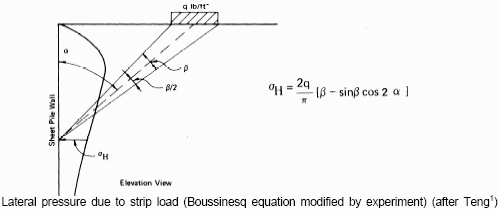Figure 1. USS (US Steel) sheet pile design manual recommendation

On the other hand the popular sheet pile design/analysis program CWALSHT by W. P. Dawkins does not apply the two factor (Figure 2). This program is distributed by US Army Engineer WES. He follows the recommendation found in the last edition of the (otherwise excellent) Foundation Engineering book by J. Bowles.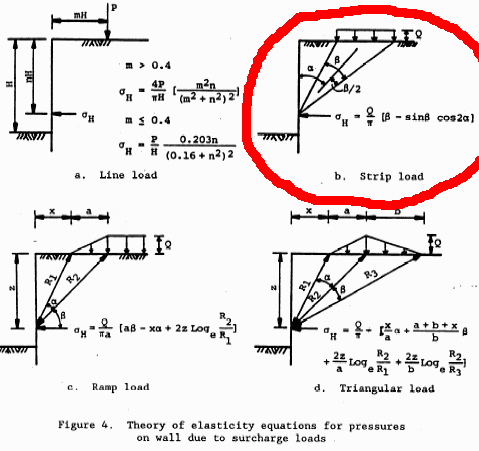Figure 2. The original elastic stresses are used by the CWALSHT without the factor of two.

All of these sources are quite reputable. So Mr. Michael Lecomte of Deerfield Beach, FL, like many other alert engineers before him, asked the question do I apply the factor of two or not?

So the question is still there. I have become familiar with it when my good friend Dr. Ergun of METU was building a huge sand box with a wall next to it covered with stress cells. He explained to me that someone reported measuring wall stresses twice as large as the elastic theory predicts. This happened way back in 20th Century in one of the early World Conferences of Soil Mechanics and Foundatiion Engineering (I think the very first one), when Mindlin (who gave us the fundamental point-load solutions for a buried force) jumped up yelling "of course" and explained it with an "imaginary mirror load" model.

Dr. Ergun found the same factor of two with his tests.

## But Why a Factor of Two?

In one of my funny T/F test questions I said "it is because the theory is so wrong, and soils are not even elastic" to which many students agreed! The fact is the theoretical solutions are for an elastic half space (i.e., infinite in both horizontal directions), not one restricted by a wall, especially so close to the load, and where we are interested in the stresses.

Consider the problem of a point load on an elastic half space Figure 1 (a) (Boussinesq problem). The theory of elasticity is based on linear differential equations. Before you start yawning, I will tell you that that is exactly what makes superposition possible: In case you forgot, a fundamental theorem is that

If a function f is a solution of a linear differential equation and another function g is independently another solution, then any linear combination of f and g

c1f + c2 g

is also a solution. This is what makes the integration of Boussinesq solution for any load shape, as well as other superpositions we routinely do, legitimate.

Now, in Figure 3, (a) shows the actual point load Q, and (b) shows another point load, Q' as Mindlin imagined. A-A is a vertical plane, at some arbitrary distance L from the load, where we consider the stresses and displacements due to these two loads.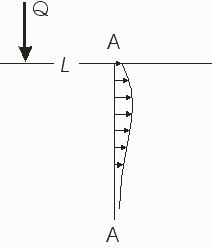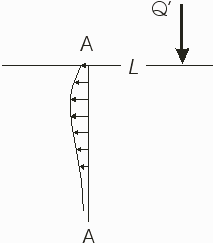(a) Displacement of the verticalplane A-A due to load Q (b) Displacement of the verticalplane A-A due to load Q'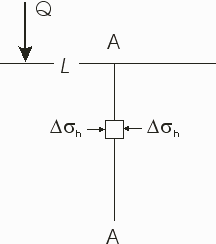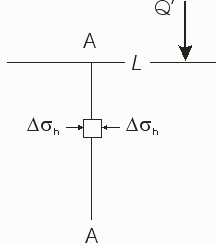(c) Stresses on the verticalplane A-A due to load Q (d) Stresses on the verticalplane A-A due to load Q'

Figure 3. The horizontal stresses and displacements over a vertical plane in
an elastic half space due to a vertical load on the surface.

Now compare the effects of the actual load Q and the imaginary mirror load Q'. Notice that

• The displacements are in the opposite direction, comparing (a) and (b), but
• Both stresses are compression, comparing (c) and (d).

This means that if the two forces were acting together, solutions could be superposed leading to a condition where the displacements would cancel each other, while the stresses would double. Therefore, if the two loads were acting together, our plane A-A would not move. It means that if we were to remove all the material from the right side of the plane A-A, while still maintaining its position, the material on the other side of the plane would not even "know" about it. To maintain the A-A plane in position though, we would have to provide the support equal to twice the stresses due to Q alone. So this represents a rigid boundary simulating a rigid retaining wall.

Again by virtue of the linearity of the differential equation as explained above, this conclusion is valid not just for a point-load, but to all other load shapes for which solutions are obtained by integration of the point-load solution.

## One Last Note

To correct the blunder in CWALSHT you have to apply the factor of two yourself, before feeding it to the program.

And if you have designed a wall with CWALSHT and it is still standing, thank God for his grace.